# TSA Real Cepstrum VI

LabVIEW 2014 Advanced Signal Processing Toolkit Help

Edition Date: June 2014

Part Number: 372656C-01

»View Product InfoDownload Help (Windows Only)

Owning Palette: Correlation and Spectral Analysis VIs

Computes the single-sided real cepstrum of a univariate time series. You can use the resulting real cepstrum to detect the periodicities of the time series. The real cepstrum does not keep the phase information of the time series, so you cannot reconstruct the original time series from the real cepstrum. Wire data to the Xt input to determine the polymorphic instance to use or manually select the instance.

Use the pull-down menu to select an instance of this VI.

 Select an instance TSA Real Cepstrum (Waveform)TSA Real Cepstrum (Array)

## TSA Real Cepstrum (Waveform)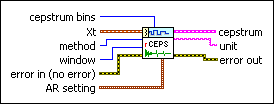cepstrum bins specifies the number of time points. This VI computes the cepstrum for each time point. The default is –1, which specifies that the number of cepstrum bins equals the length of the input time series.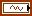Xt specifies the univariate time series.method specifies whether this VI uses the FFT-based or the autoregressive (AR) model-based method to compute the real cepstrum. The default is FFT. Refer to the Details section for more information about each method.window specifies the time-domain window applied to the time series. Options include None (default), Hanning, Hamming, Blackman-Harris, Exact Blackman, Blackman, Flat Top, 4 Term B-Harris, 7 Term B-Harris, and Low Sidelobe.error in describes error conditions that occur before this node runs. This input provides standard error in functionality.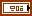AR setting specifies the settings for the autoregressive (AR) model. This option is valid only when the method is AR Model.AR method specifies the method this VI uses to estimate the autoregressive (AR) model.

 0 Forward-Backward (default)—Computes the AR coefficients by minimizing the least-square errors of the forward and backward predictions. 1 Least-Squares—Computes the AR coefficients by minimizing the least-square errors of the forward predictions. 2 Yule-Walker—Computes the AR coefficients by solving the Yule-Walker functions based on the forward predictions. 3 Burg-Lattice—Computes the AR coefficients using the Levinson-Durbin recursion based on the forward and backward predictions. The Levinson-Durbin recursion uses the arithmetic average. 4 Geometric-Lattice—Computes the AR coefficients using the Levinson-Durbin recursion based on the forward and backward predictions. The Levinson-Durbin recursion uses the geometric average.AR order specifies the order of the autoregressive (AR) model. The value of AR order must be greater than 0. The default is 4.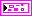cepstrum returns the cepstrum information about Xt.t0 returns the lower boundary of the time range.dt returns the time increment.C(t) returns the resulting values of the complex cepstrum.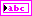unit returns the engineering unit of the PSD. You can specify an engineering unit for a time series by using the TSA Scale to EU VI.error out contains error information. This output provides standard error out functionality.

## TSA Real Cepstrum (Array)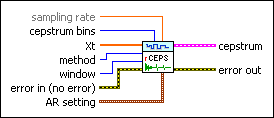sampling rate specifies the sampling rate, in hertz, of the univariate time series Xt. The default is 1.cepstrum bins specifies the number of time points. This VI computes the cepstrum for each time point. The default is –1, which specifies that the number of cepstrum bins equals the length of the input time series.Xt specifies the univariate time series.method specifies whether this VI uses the FFT-based or the autoregressive (AR) model-based method to compute the real cepstrum. The default is FFT. Refer to the Details section for more information about each method.window specifies the time-domain window applied to the time series. Options include None (default), Hanning, Hamming, Blackman-Harris, Exact Blackman, Blackman, Flat Top, 4 Term B-Harris, 7 Term B-Harris, and Low Sidelobe.error in describes error conditions that occur before this node runs. This input provides standard error in functionality.AR setting specifies the settings for the autoregressive (AR) model. This option is valid only when the method is AR Model.AR method specifies the method this VI uses to estimate the autoregressive (AR) model.

 0 Forward-Backward (default)—Computes the AR coefficients by minimizing the least-square errors of the forward and backward predictions. 1 Least-Squares—Computes the AR coefficients by minimizing the least-square errors of the forward predictions. 2 Yule-Walker—Computes the AR coefficients by solving the Yule-Walker functions based on the forward predictions. 3 Burg-Lattice—Computes the AR coefficients using the Levinson-Durbin recursion based on the forward and backward predictions. The Levinson-Durbin recursion uses the arithmetic average. 4 Geometric-Lattice—Computes the AR coefficients using the Levinson-Durbin recursion based on the forward and backward predictions. The Levinson-Durbin recursion uses the geometric average.AR order specifies the order of the autoregressive (AR) model. The value of AR order must be greater than 0. The default is 4.cepstrum returns the cepstrum information about Xt.t0 returns the lower boundary of the time range.dt returns the time increment.C(t) returns the resulting values of the complex cepstrum.error out contains error information. This output provides standard error out functionality.

## TSA Real Cepstrum Details

When method is FFT, this VI computes the real cepstrum of a univariate time series according to the following equation: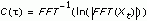Xt is the univariate time series and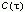is the real cepstrum of Xt.

When method is AR Model, this VI computes the real cepstrum of a univariate time series according to the following equation:s is the standard deviation of estimated noise series of AR model of Xt and a is the estimated coefficients of AR model. a = [1, a1, a2, …, ak].

## Examples

Refer to the following VIs for examples of using the TSA Real Cepstrum VI:

• Bearing Monitor VI: labview\examples\Time Series Analysis\TSAApplications
• Cepstrum Analysis VI: labview\examples\Time Series Analysis\TSAGettingStarted